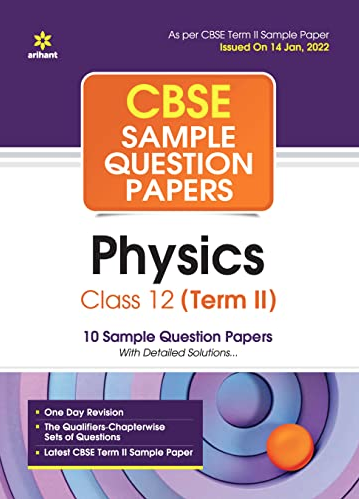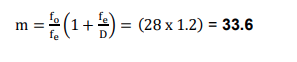# (a) Draw a ray diagram of Astronomical Telescope for the final image formed at infinity. (b) A small telescope has an objective lens of focal length 140 cm and an eyepiece of focal length 5.0 cm. Find the magnifying power of the telescope for viewing distant objects when (i) the telescope is in normal adjustment, (ii) the final image is formed at the least distance of distinct vision.

CBSE Sample Question Paper, Class 12 Physics Term 2 Question - (a) Draw a ray diagram of Astronomical Telescope for the final image formed at infinity. (b) A small telescope has an objective lens of focal length 140 cm and an eyepiece of focal length 5.0 cm. Find the magnifying power of the telescope for viewing distant objects when (i) the telescope is in normal adjustment, (ii) the final image is formed at the least distance of distinct vision.## (a) Draw a ray diagram of Astronomical Telescope for the final image formed at infinity. (b) A small telescope has an objective lens of focal length 140 cm and an eyepiece of focal length 5.0 cm. Find the magnifying power of the telescope for viewing distant objects when (i) the telescope is in normal adjustment, (ii) the final image is formed at the least distance of distinct vision.

### (b) (i) In normal adjustment : Magnifying power. m = f୭/fୣ = (140/5) = 28 (ii) When the final image is formed at the least distance of distinct vision (25 cm) :